International
Tables for
Crystallography
Volume B
Reciprocal space
Edited by U. Shmueli

International Tables for Crystallography (2006). Vol. B, ch. 1.3, pp. 29-30   | 1 | 2 |

Section 1.3.2.3.3. Test-function spaces

G. Bricognea

aMRC Laboratory of Molecular Biology, Hills Road, Cambridge CB2 2QH, England, and LURE, Bâtiment 209D, Université Paris-Sud, 91405 Orsay, France

1.3.2.3.3. Test-function spaces

| top | pdf |

With this rationale in mind, the following function spaces will be defined for any open subset Ω of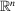(which may be the whole of):

 (a)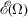is the space of complex-valued functions over Ω which are indefinitely differentiable; (b)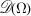is the subspace ofconsisting of functions with (unspecified) compact support contained in; (c)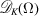is the subspace ofconsisting of functions whose (compact) support is contained within a fixed compact subset K of Ω.

When Ω is unambiguously defined by the context, we will simply write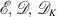.

It sometimes suffices to require the existence of continuous derivatives only up to finite order m inclusive. The corresponding spaces are then denoted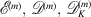with the convention that if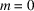, only continuity is required.

The topologies on these spaces constitute the most important ingredients of distribution theory, and will be outlined in some detail.

1.3.2.3.3.1. Topology on| top | pdf |

It is defined by the family of semi-norms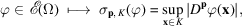where p is a multi-index and K a compact subset of Ω. A fundamental system S of neighbourhoods of the origin inis given by subsets ofof the form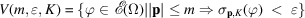for all natural integers m, positive real, and compact subset K of Ω. Since a countable family of compact subsets K suffices to cover Ω, and since restricted values ofof the form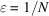lead to the same topology, S is equivalent to a countable system of neighbourhoods and henceis metrizable.

Convergence inmay thus be defined by means of sequences. A sequence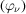inwill be said to converge to 0 if for any given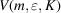there exists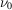such that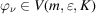whenever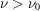; in other words, if theand all their derivatives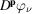converge to 0 uniformly on any given compact K in Ω.

1.3.2.3.3.2. Topology on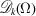| top | pdf |

It is defined by the family of semi-norms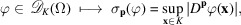where K is now fixed. The fundamental system S of neighbourhoods of the origin in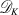is given by sets of the form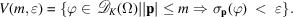It is equivalent to the countable subsystem of the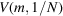, henceis metrizable.

Convergence inmay thus be defined by means of sequences. A sequenceinwill be said to converge to 0 if for any given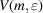there existssuch that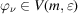whenever; in other words, if theand all their derivativesconverge to 0 uniformly in K.

1.3.2.3.3.3. Topology on| top | pdf |

It is defined by the fundamental system of neighbourhoods of the origin consisting of sets of the form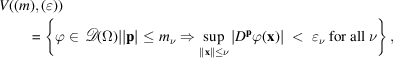where (m) is an increasing sequence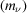of integers tending to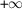and () is a decreasing sequence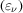of positive reals tending to 0, as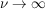.

This topology is not metrizable, because the sets of sequences (m) and () are essentially uncountable. It can, however, be shown to be the inductive limit of the topology of the subspaces, in the following sense: V is a neighbourhood of the origin in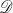if and only if its intersection withis a neighbourhood of the origin infor any given compact K in Ω.

A sequenceinwill thus be said to converge to 0 inif all thebelong to some(with K a compact subset of Ω independent of ν) and ifconverges to 0 in.

As a result, a complex-valued functional T onwill be said to be continuous for the topology ofif and only if, for any given compact K in Ω, its restriction tois continuous for the topology of, i.e. maps convergent sequences into convergent sequences in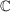.

This property of, i.e. having a non-metrizable topology which is the inductive limit of metrizable topologies in its subspaces, conditions the whole structure of distribution theory and dictates that of many of its proofs.

1.3.2.3.3.4. Topologies on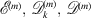| top | pdf |

These are defined similarly, but only involve conditions on derivatives up to order m.